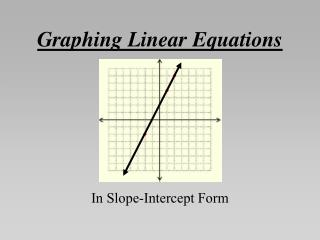DownloadDownload PresentationGraphing Linear Equations

# Graphing Linear Equations

Télécharger la présentation## Graphing Linear Equations

- - - - - - - - - - - - - - - - - - - - - - - - - - - E N D - - - - - - - - - - - - - - - - - - - - - - - - - - -
##### Presentation Transcript

1. Graphing Linear Equations In Slope-Intercept Form

2. We have already seen that linear equations have two variables and when we plot all the (x,y) pairs that make the equation true we get a line. In this section, instead of making a table, evaluating y for each x, plotting the points and making a line, we will use The Slope-Intercept Form of the equation to graph the line.

3. These equations are all in Slope-Intercept Form: Notice that these equations are all solved for y.

4. Just by looking at an equation in this form, we can draw the line (no tables). • The constant is the y-intercept. • The coefficient is the slope. Constant = 1, y-intercept = 1. Coefficient = 2, slope = 2. Constant = -4, y-intercept = -4. Coefficient = -1, slope = -1. Constant = -2, y-intercept = -2. Coefficient = 3/2, slope = 3/2.

5. The formula for Slope-Intercept Form is: • ‘b’ is the y-intercept. • ‘m’ is the slope. On the next three slides we will graph the three equations: using their y-intercepts and slopes.

6. right 1 up 2 right 1 up 2 1) Plot the y-intercept as a point on the y-axis. The constant, b = 1, so the y-intercept = 1. 2) Plot more points by counting the slope up the numerator (down if negative) and right the denominator. The coefficient, m = 2, so the slope = 2/1.

7. down 1 down 1 right 1 right 1 1) Plot the y-intercept as a point on the y-axis. The constant, b = -4, so the y-intercept = -4. 2) Plot more points by counting the slope up the numerator (down if negative) and right the denominator. The coefficient, m = -1, so the slope = -1/1.

8. right 2 up 3 right 2 up 3 1) Plot the y-intercept as a point on the y-axis. The constant, b = -2, so the y-intercept = -2. 2) Plot more points by counting the slope up the numerator (down if negative) and right the denominator. The coefficient, m = 3/2, so the slope = 3/2.

9. Sometimes we must solve the equation for y before we can graph it. The constant, b = 3 is the y-intercept. The coefficient, m = -2 is the slope.

10. down 2 down 2 right 1 right 1 1) Plot the y-intercept as a point on the y-axis. The constant, b = 3, so the y-intercept = 3. 2) Plot more points by counting the slope up the numerator (down if negative) and right the denominator. The coefficient, m = -2, so the slope = -2/1.

11. Graphing more than one Line on the same graph y= 1/3x + 3 y = 1/3x + 3/2

12. What did you notice about the two lines on the previous slide? • Parallel Lines are lines that don’t intersect 2x + 3y = 6 y = 2/3x + 4

13. Classwork/Homework